# 一、从目的出发

## 1.导入数据

• sepallength：萼片长度
• sepalwidth：萼片宽度
• petallength：花瓣长度
• petalwidth：花瓣宽度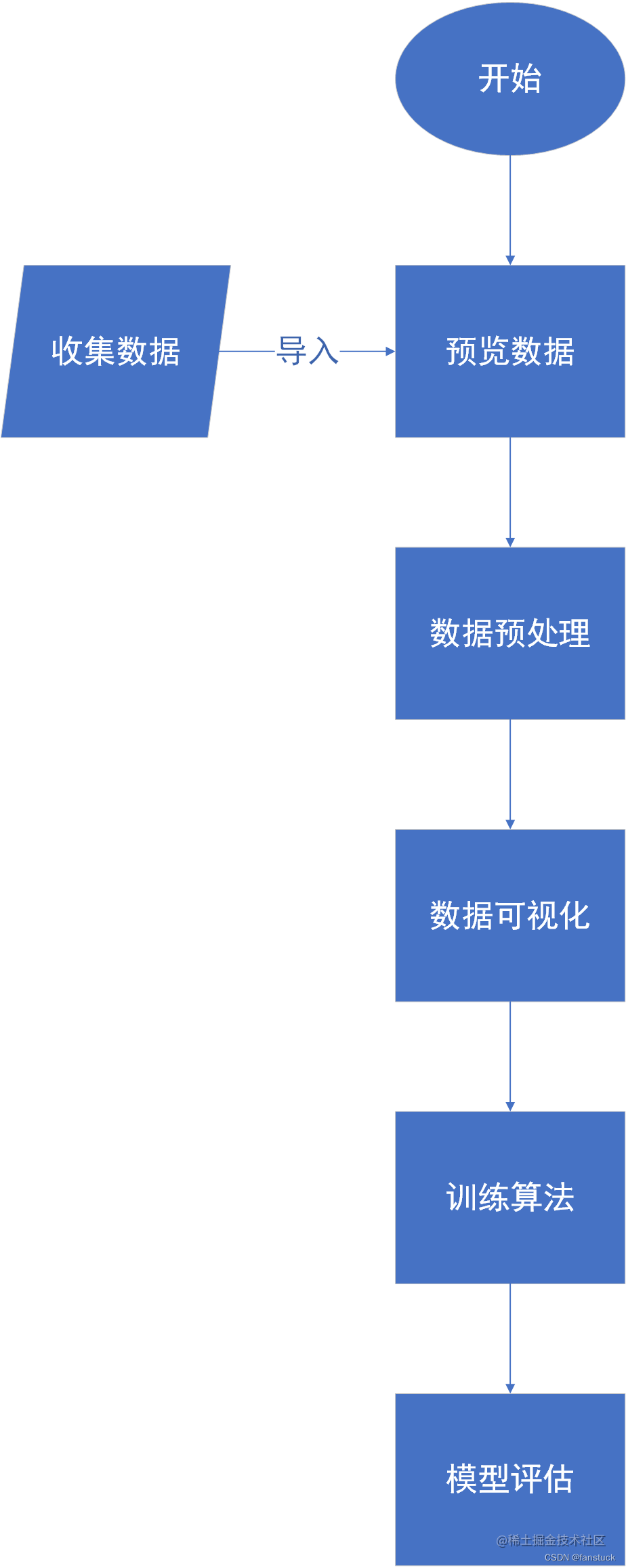# 二、项目开启

## 1.导入数据

```from sklearn.datasets import load_iris import pandas as pd``` 之后只需要登陆数据就可以看到数据的形式了：

```iris_data = load_iris() iris_data_feature=list(iris_data.data) iris_data_df=pd.DataFrame(iris_data_feature,columns=['花萼长度','花萼宽度','花瓣长度','花瓣宽度']) iris_data_df```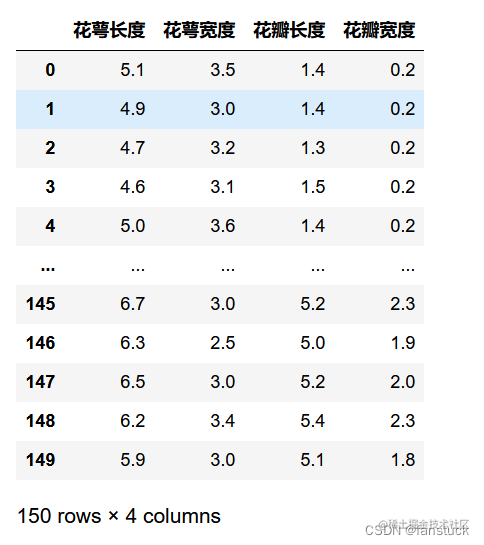```iris_data_class=list(iris_data.target) iris_class_df=pd.DataFrame(iris_data_class,columns=['花朵类型']) iris_class_df```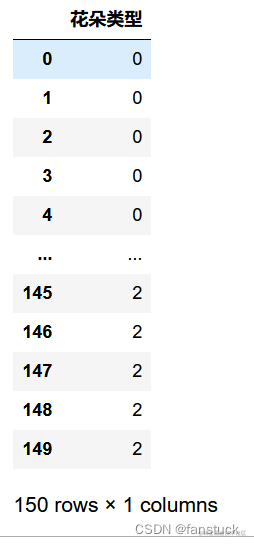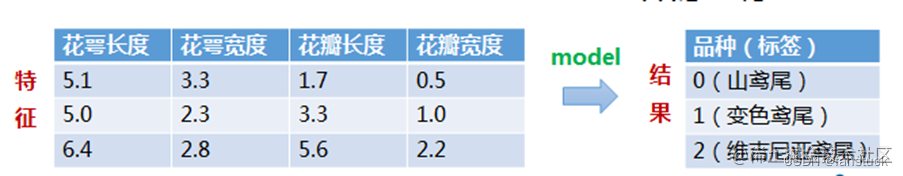`iris_true_df=pd.concat([iris_data_df,iris_class_df],axis=1)`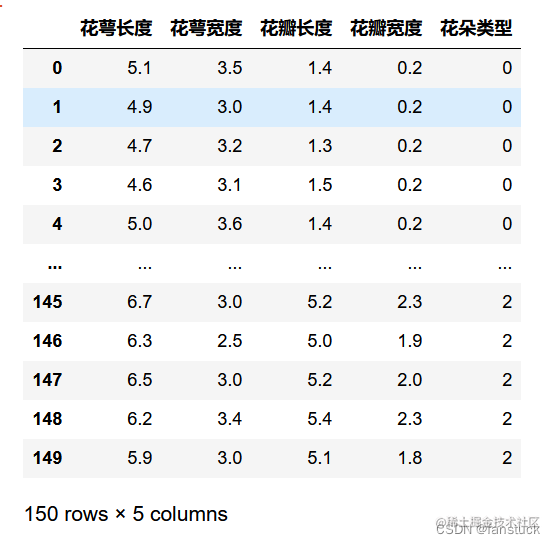## 2.预览数据

`iris_true_df.describe()`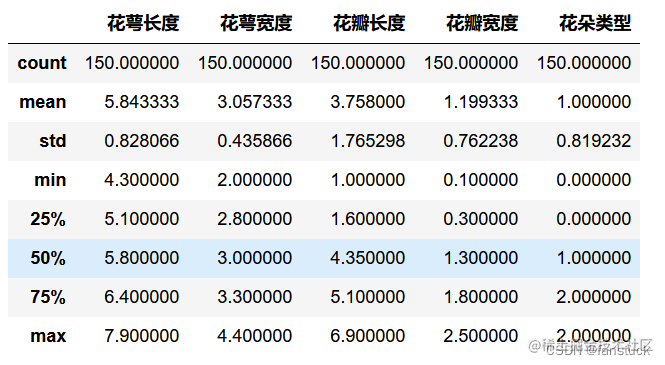• count：数量统计，此列共有多少有效值
• unipue：不同的值有多少个
• std：标准差

• min：最小值

• 25%：四分之一分位数

• 50%：二分之一分位数

• 75%：四分之三分位数

• max：最大值

• mean：均值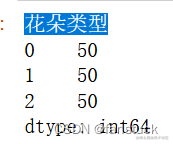```iris_true_gy=iris_true_df.groupby('花朵类型') for name,group in iris_true_gy: print(name) display(group.head())```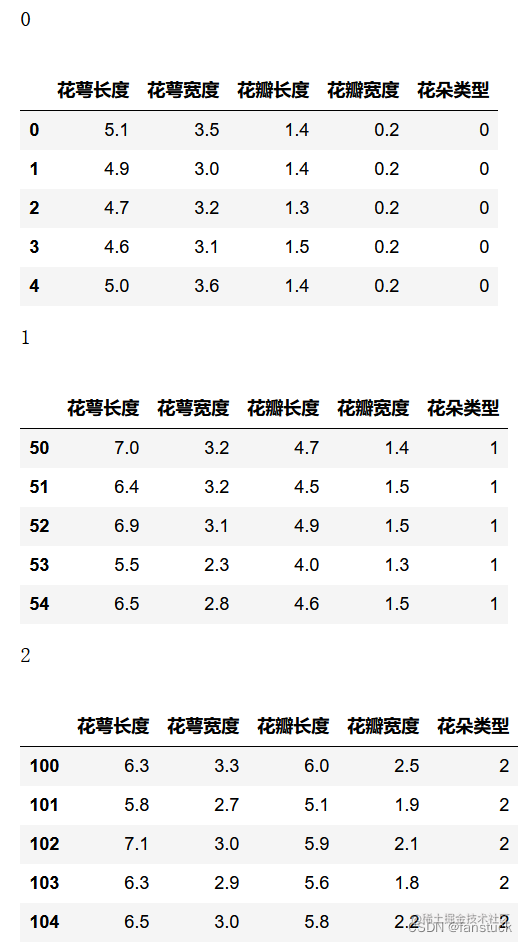`iris_true_gy['花萼长度'].agg(['min','mean','max'])`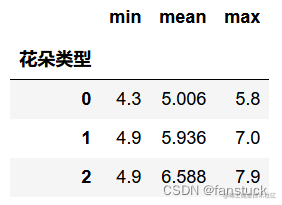## 4.数据可视化

```import matplotlib.pyplot as plt from matplotlib import font_manager # 导入字体管理模块 plt.style.use('ggplot')```

### 单变量图表

``` from pylab import mpl

# mpl.rcParams['font.sans-serif'] = ['SimHei']

mpl.rcParams['font.sans-serif'] = ['Microsoft YaHei'] # 指定默认字体：解决plot不能显示中文问题

mpl.rcParams['axes.unicode_minus'] = False # 解决保存图像是负号'-'显示为方块的问题 iris_data_df.plot(kind='box',subplots=True,layout=(2,2)) ```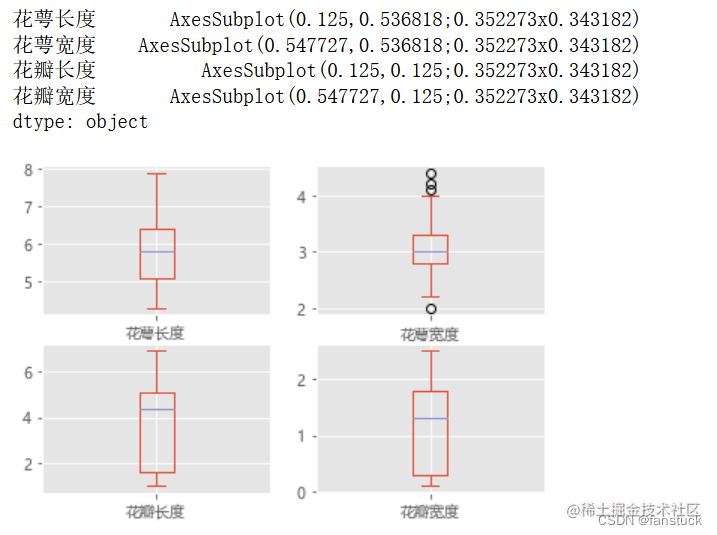`iris_data_df.hist()`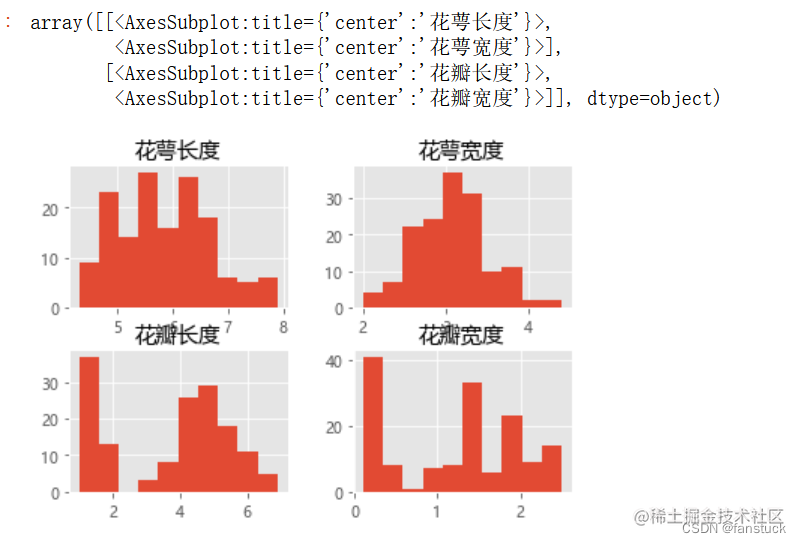```

# 散点矩阵图

scatter_matrix(iris_data_df) ```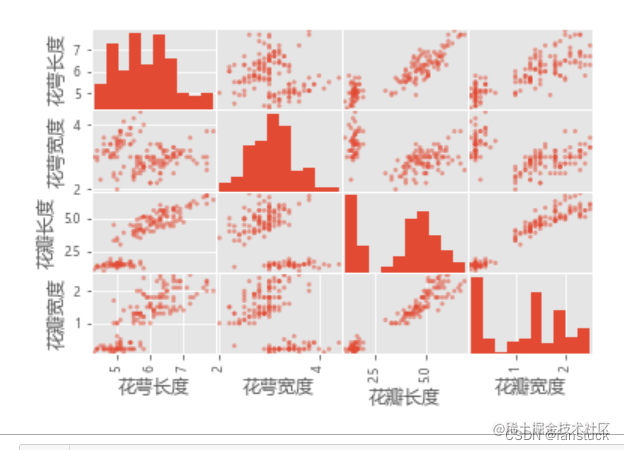```

## 第一步，先定义1张空白的大画板

fig=plt.figure(num=1, figsize=(16, 16))

## 增加1个子图，2x2，共4个子图，排第4个

ax4 = fig.add_subplot(224) ax4.scatter(x=iris_true_df.iloc[:,3:4], y=iris_true_df.iloc[:,4:5],color='c',alpha=0.5) ```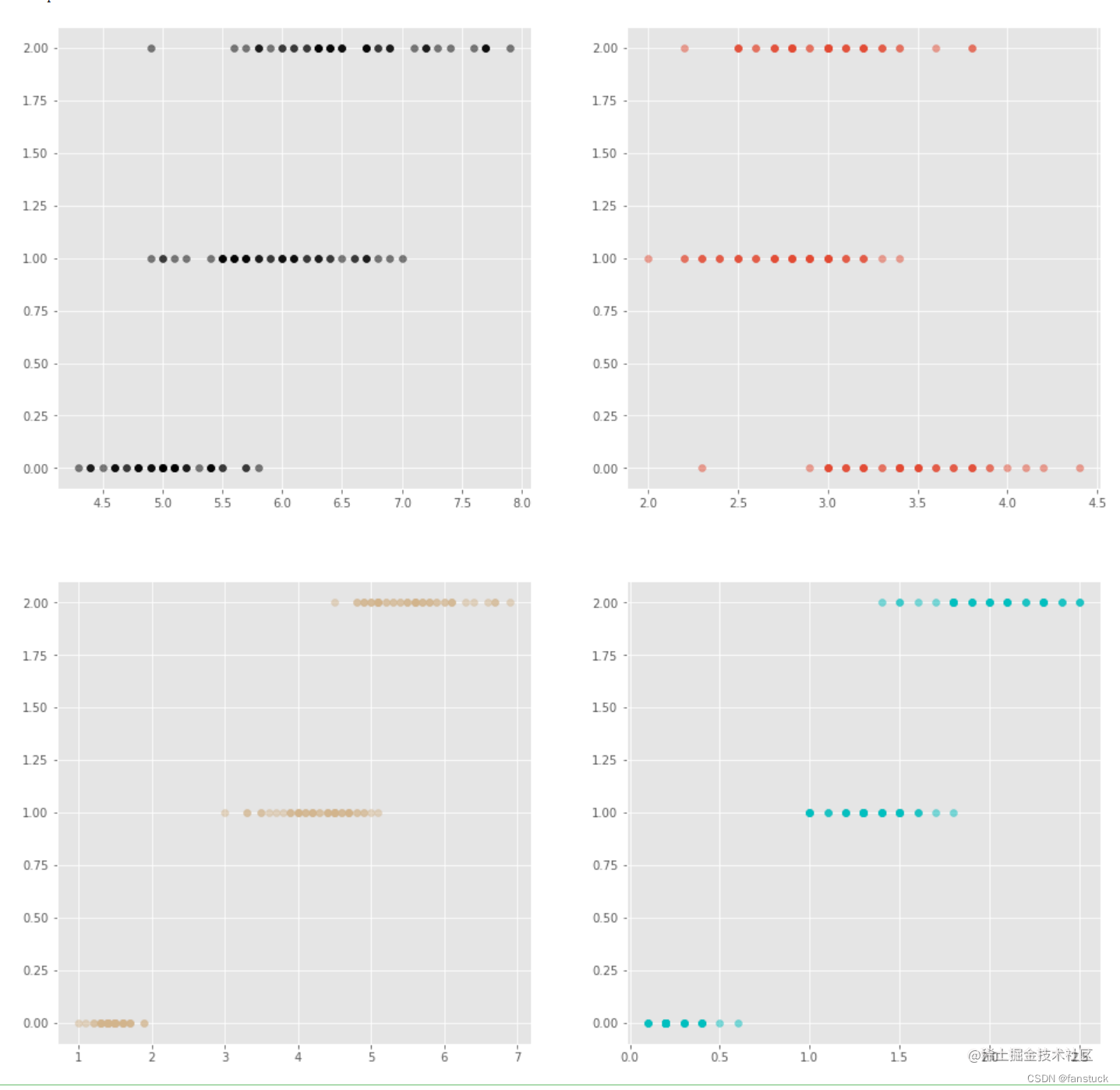## 5.训练模型

### 5.1划分数据集

```from sklearn.model_selection import train_test_split iris_array=iris_true_df.values X=iris_array[:,0:4] Y=iris_array[:,4] test_model=0.2 seed=5 X_train,X_test,Y_train,Y_test=train_test_split(X,Y,test_size=test_model,random_state=seed)```

### 5.2评估算法

`from sklearn.model_selection import KFold`

KFold和K-折验证是一样的。也就是将一份数据集划分成为K份，拿其中的一份用作验证，剩下的k-1份数据用作训练。因此，该KFold这个类也不难理解，也是起数据划分之用。

### 5.3模型建立

```from sklearn.linear_model import LogisticRegression from sklearn.tree import DecisionTreeClassifier from sklearn.discriminant_analysis import LinearDiscriminantAnalysis from sklearn.neighbors import KNeighborsClassifier from sklearn.naive_bayes import GaussianNB from sklearn.svm import SVC```  然后构建六种不同的模型：

```models={} models['LR']=LogisticRegression() models['LDA']=LinearDiscriminantAnalysis() models['KNN']=KNeighborsClassifier() models['CART']=DecisionTreeClassifier() models['NB']=GaussianNB() models['SVM']=SVC()```  之后我需要建立模型评估算法：

```results=[] for key in models: kfold=KFold(n_splits=10,shuffle=True,random_state=seed) cv_results=cross_val_score(models[key],X_train,Y_train,cv=kfold,scoring='accuracy') results.append(cv_results) print('%s:%f(%f)'%(key,cv_results.mean(),cv_results.std()))```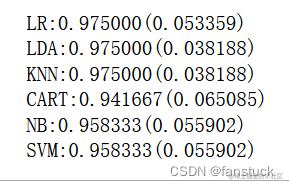```

# 箱线图

fig=pyplot.figure() fig.suptitle('Comparison') ax = fig.add_subplot(111) pyplot.boxplot(results) ax.set_xticklabels(models.keys()) pyplot.show() ```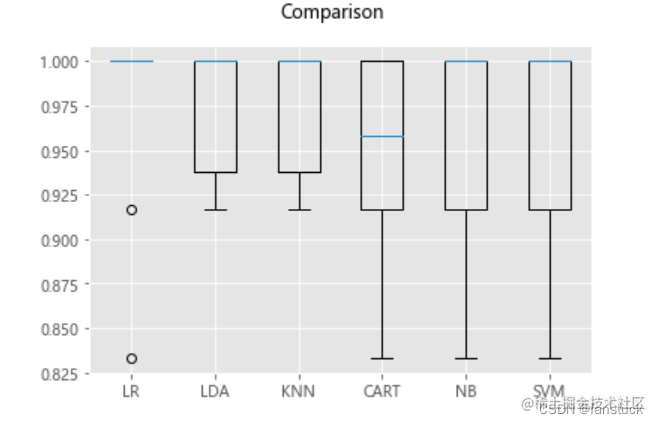### 5.4模型预测

```LR=LogisticRegression() LR.fit(X=X_train,y=Y_train) predictions=LR.predict(X_test) print(accuracy_score(Y_test,predictions)) print(confusion_matrix(Y_test,predictions)) print(classification_report(Y_test,predictions))```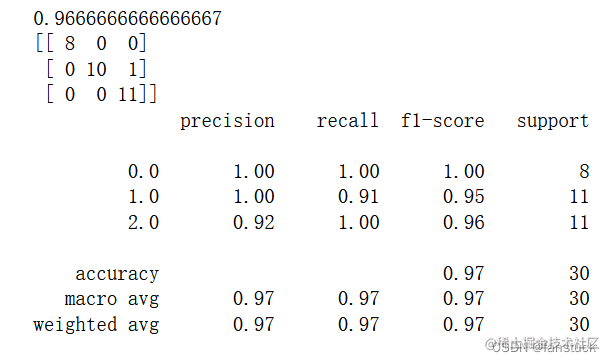confusion_matrix为混淆矩阵。classification_report提供了每个类别的预测情况，精准率，召回率，F1。